Publicité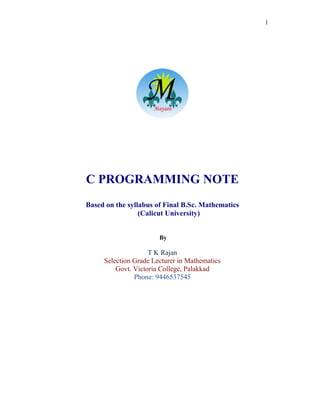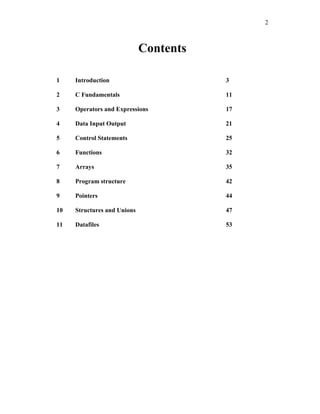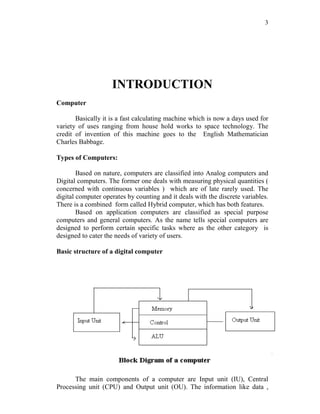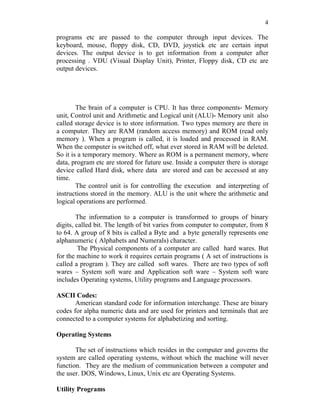Publicité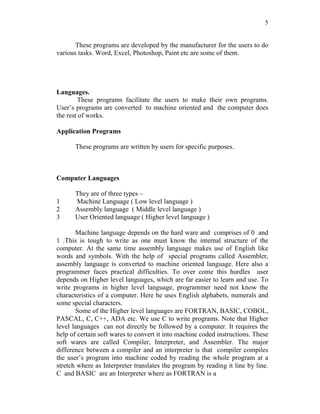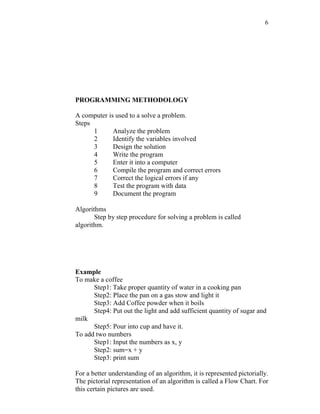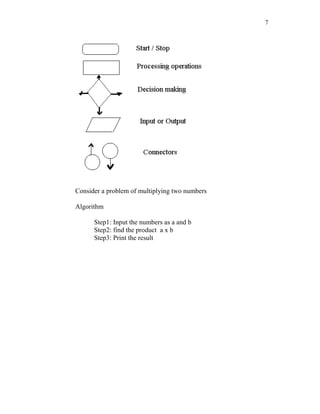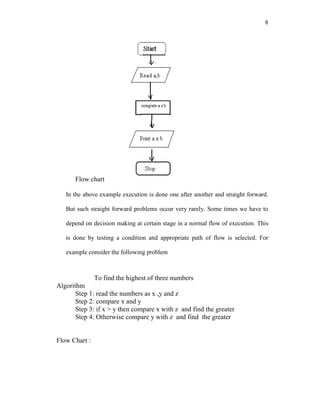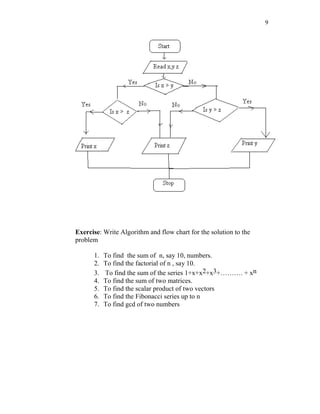Publicité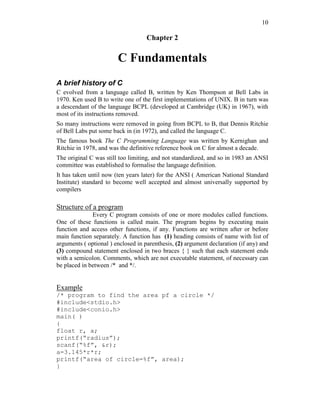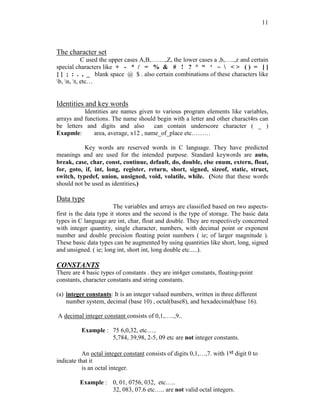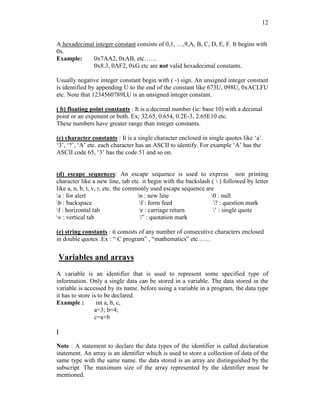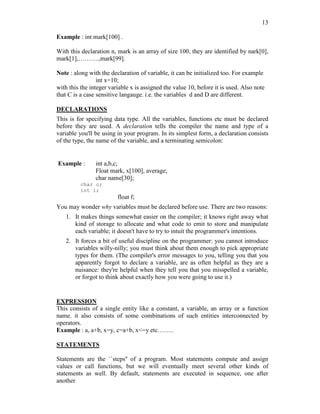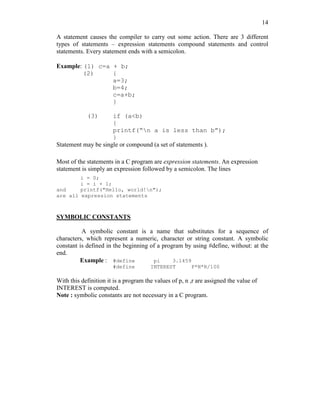Publicité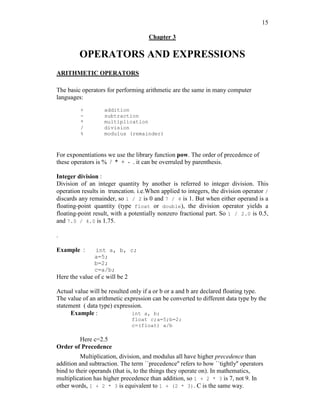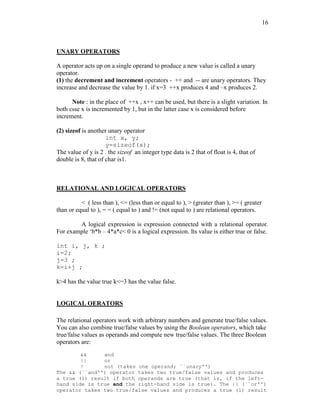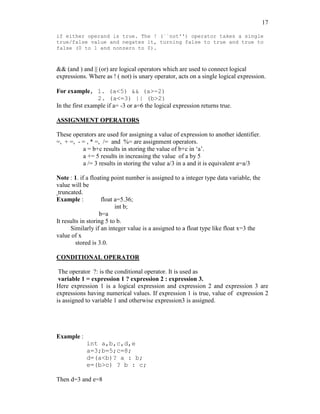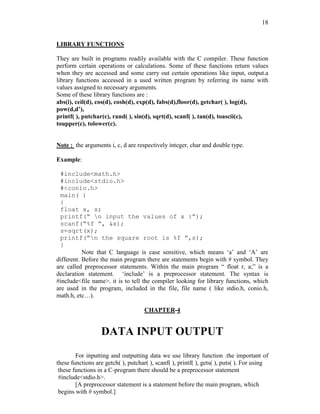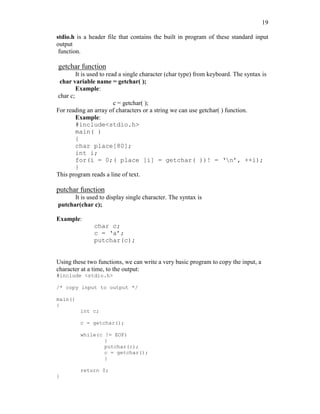Publicité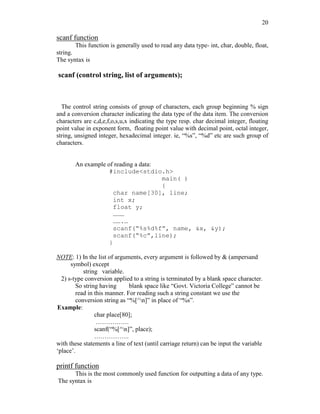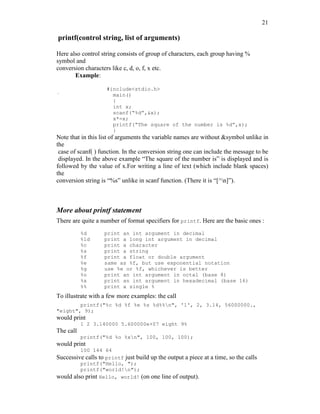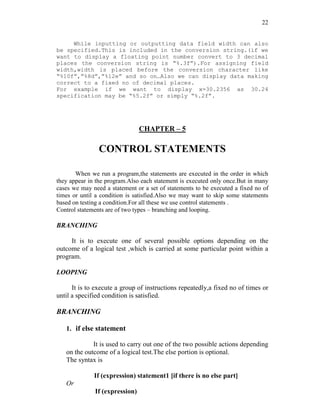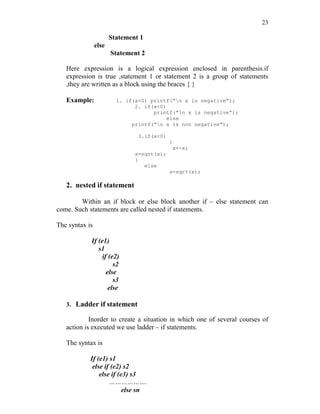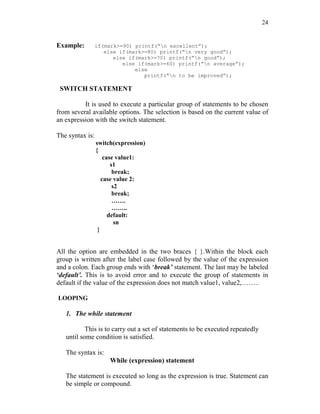Publicité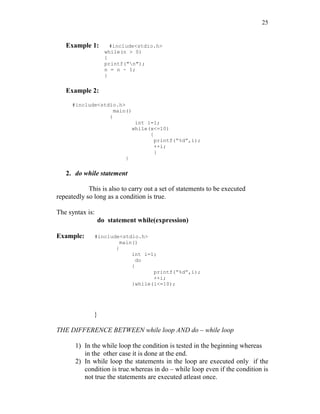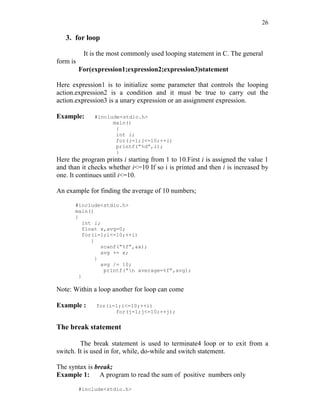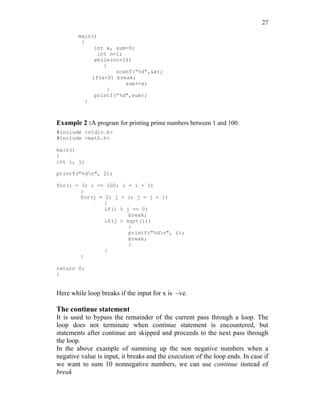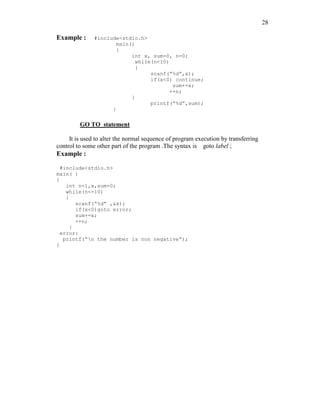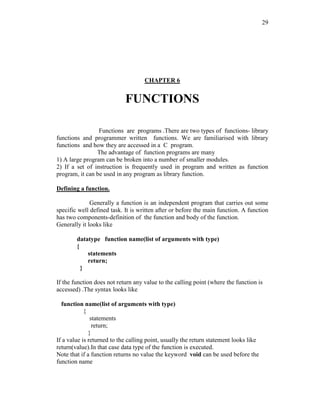Publicité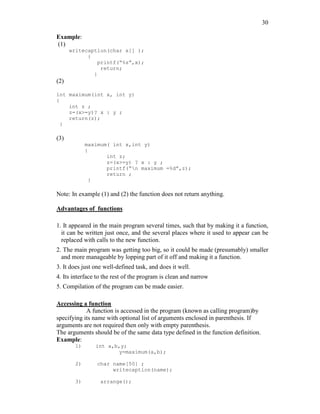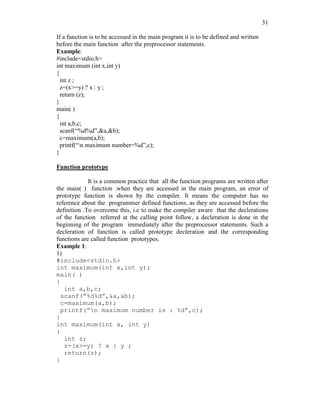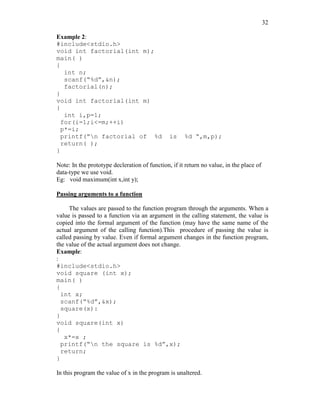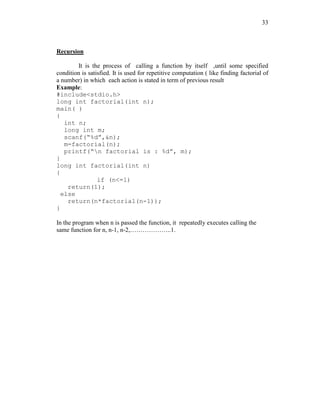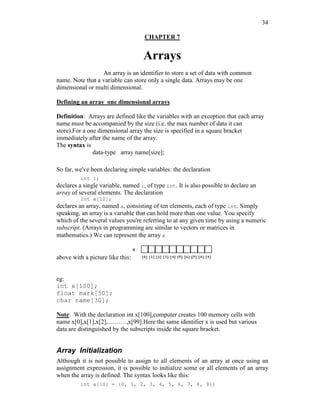Publicité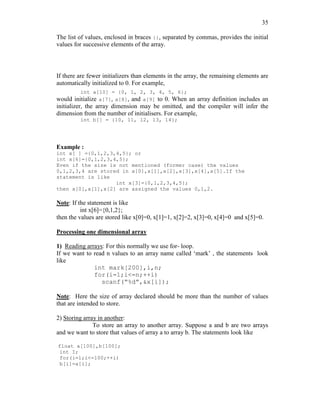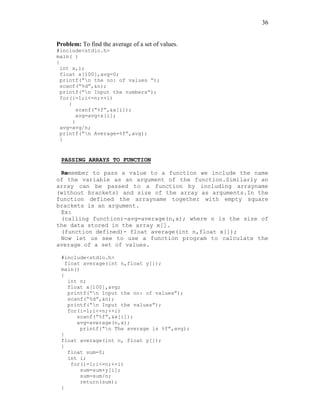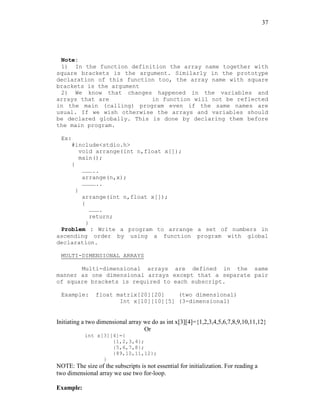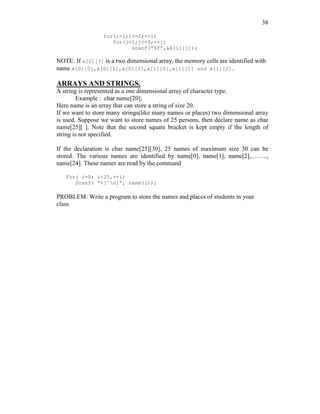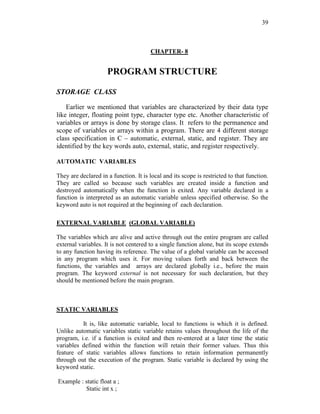Publicité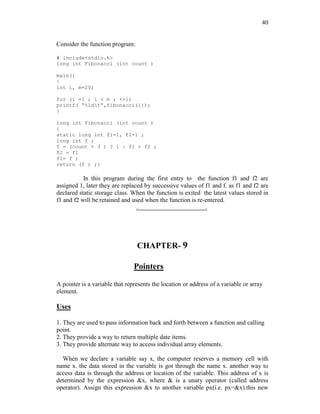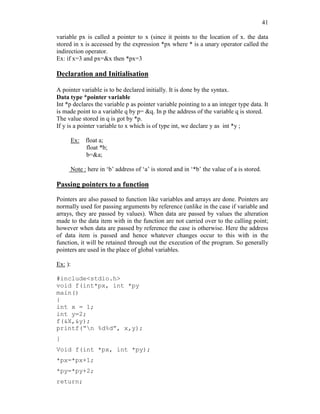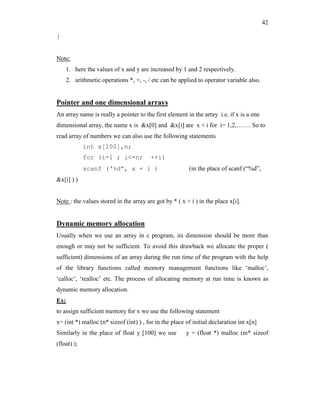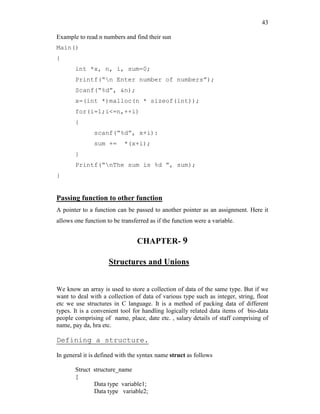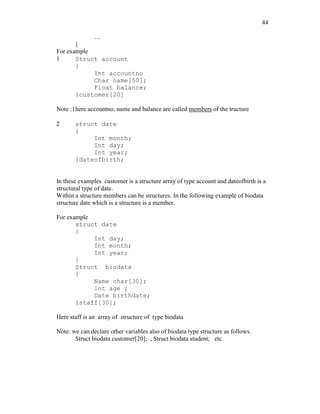Publicité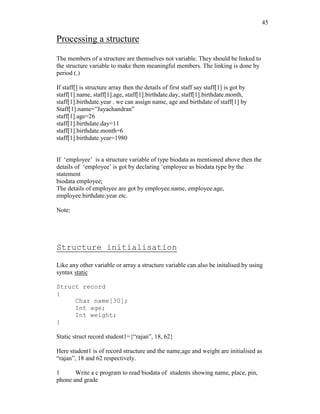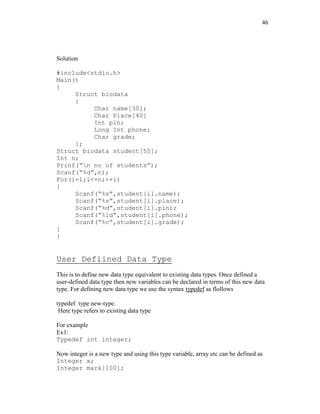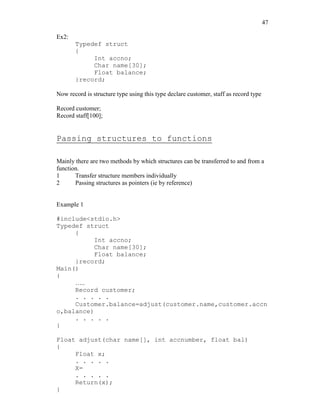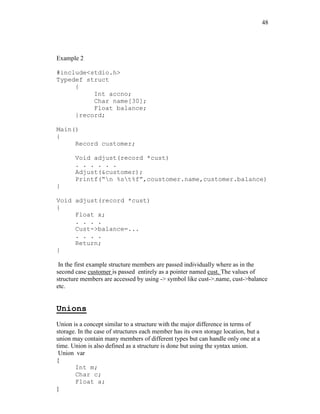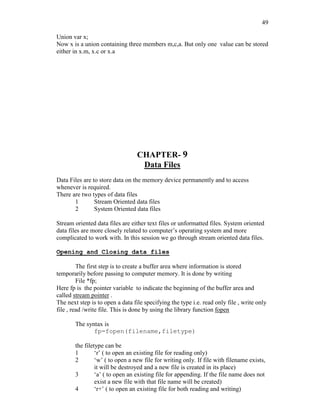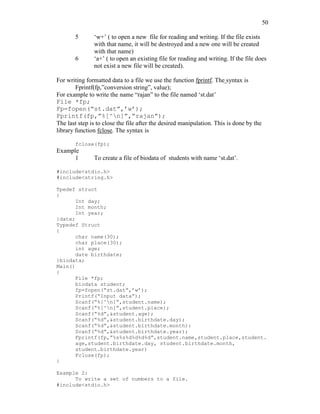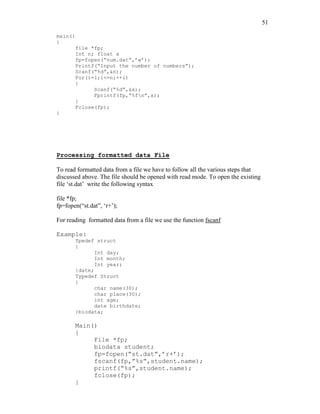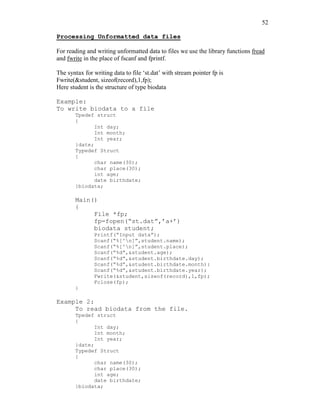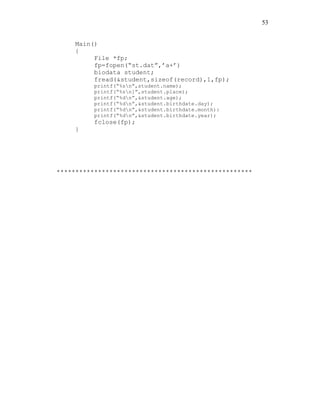Prochain SlideShare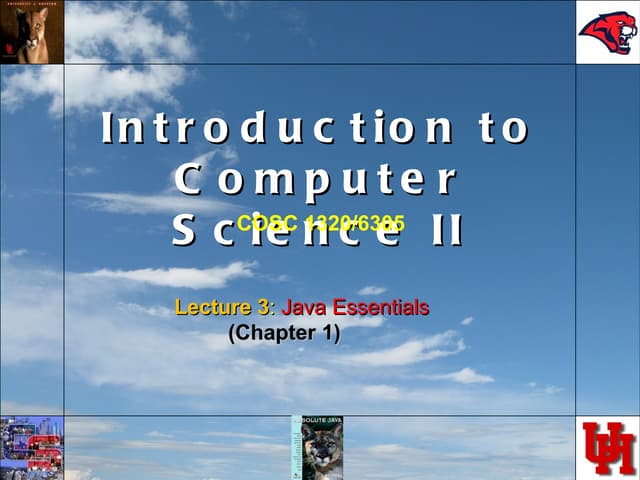Lecture 3 java basics
Chargement dans ... 3
1 sur 53
Publicité

### C progrmming

1. 1 C PROGRAMMING NOTE Based on the syllabus of Final B.Sc. Mathematics (Calicut University) By T K Rajan Selection Grade Lecturer in Mathematics Govt. Victoria College, Palakkad Phone: 9446537545
2. 2 Contents 1 Introduction 3 2 C Fundamentals 11 3 Operators and Expressions 17 4 Data Input Output 21 5 Control Statements 25 6 Functions 32 7 Arrays 35 8 Program structure 42 9 Pointers 44 10 Structures and Unions 47 11 Datafiles 53
3. 3 INTRODUCTION Computer Basically it is a fast calculating machine which is now a days used for variety of uses ranging from house hold works to space technology. The credit of invention of this machine goes to the English Mathematician Charles Babbage. Types of Computers: Based on nature, computers are classified into Analog computers and Digital computers. The former one deals with measuring physical quantities ( concerned with continuous variables ) which are of late rarely used. The digital computer operates by counting and it deals with the discrete variables. There is a combined form called Hybrid computer, which has both features. Based on application computers are classified as special purpose computers and general computers. As the name tells special computers are designed to perform certain specific tasks where as the other category is designed to cater the needs of variety of users. Basic structure of a digital computer The main components of a computer are Input unit (IU), Central Processing unit (CPU) and Output unit (OU). The information like data ,
4. 4 programs etc are passed to the computer through input devices. The keyboard, mouse, floppy disk, CD, DVD, joystick etc are certain input devices. The output device is to get information from a computer after processing . VDU (Visual Display Unit), Printer, Floppy disk, CD etc are output devices. The brain of a computer is CPU. It has three components- Memory unit, Control unit and Arithmetic and Logical unit (ALU)- Memory unit also called storage device is to store information. Two types memory are there in a computer. They are RAM (random access memory) and ROM (read only memory ). When a program is called, it is loaded and processed in RAM. When the computer is switched off, what ever stored in RAM will be deleted. So it is a temporary memory. Where as ROM is a permanent memory, where data, program etc are stored for future use. Inside a computer there is storage device called Hard disk, where data are stored and can be accessed at any time. The control unit is for controlling the execution and interpreting of instructions stored in the memory. ALU is the unit where the arithmetic and logical operations are performed. The information to a computer is transformed to groups of binary digits, called bit. The length of bit varies from computer to computer, from 8 to 64. A group of 8 bits is called a Byte and a byte generally represents one alphanumeric ( Alphabets and Numerals) character. The Physical components of a computer are called hard wares. But for the machine to work it requires certain programs ( A set of instructions is called a program ). They are called soft wares. There are two types of soft wares – System soft ware and Application soft ware – System soft ware includes Operating systems, Utility programs and Language processors. ASCII Codes: American standard code for information interchange. These are binary codes for alpha numeric data and are used for printers and terminals that are connected to a computer systems for alphabetizing and sorting. Operating Systems The set of instructions which resides in the computer and governs the system are called operating systems, without which the machine will never function. They are the medium of communication between a computer and the user. DOS, Windows, Linux, Unix etc are Operating Systems. Utility Programs
5. 5 These programs are developed by the manufacturer for the users to do various tasks. Word, Excel, Photoshop, Paint etc are some of them. Languages. These programs facilitate the users to make their own programs. User’s programs are converted to machine oriented and the computer does the rest of works. Application Programs These programs are written by users for specific purposes. Computer Languages They are of three types – 1 Machine Language ( Low level language ) 2 Assembly language ( Middle level language ) 3 User Oriented language ( Higher level language ) Machine language depends on the hard ware and comprises of 0 and 1 .This is tough to write as one must know the internal structure of the computer. At the same time assembly language makes use of English like words and symbols. With the help of special programs called Assembler, assembly language is converted to machine oriented language. Here also a programmer faces practical difficulties. To over come this hurdles user depends on Higher level languages, which are far easier to learn and use. To write programs in higher level language, programmer need not know the characteristics of a computer. Here he uses English alphabets, numerals and some special characters. Some of the Higher level languages are FORTRAN, BASIC, COBOL, PASCAL, C, C++, ADA etc. We use C to write programs. Note that Higher level languages can not directly be followed by a computer. It requires the help of certain soft wares to convert it into machine coded instructions. These soft wares are called Compiler, Interpreter, and Assembler. The major difference between a compiler and an interpreter is that compiler compiles the user’s program into machine coded by reading the whole program at a stretch where as Interpreter translates the program by reading it line by line. C and BASIC are an Interpreter where as FORTRAN is a
6. 6 PROGRAMMING METHODOLOGY A computer is used to a solve a problem. Steps 1 Analyze the problem 2 Identify the variables involved 3 Design the solution 4 Write the program 5 Enter it into a computer 6 Compile the program and correct errors 7 Correct the logical errors if any 8 Test the program with data 9 Document the program Algorithms Step by step procedure for solving a problem is called algorithm. Example To make a coffee Step1: Take proper quantity of water in a cooking pan Step2: Place the pan on a gas stow and light it Step3: Add Coffee powder when it boils Step4: Put out the light and add sufficient quantity of sugar and milk Step5: Pour into cup and have it. To add two numbers Step1: Input the numbers as x, y Step2: sum=x + y Step3: print sum For a better understanding of an algorithm, it is represented pictorially. The pictorial representation of an algorithm is called a Flow Chart. For this certain pictures are used.
7. 7 Consider a problem of multiplying two numbers Algorithm Step1: Input the numbers as a and b Step2: find the product a x b Step3: Print the result
8. 8 Flow chart In the above example execution is done one after another and straight forward. But such straight forward problems occur very rarely. Some times we have to depend on decision making at certain stage in a normal flow of execution. This is done by testing a condition and appropriate path of flow is selected. For example consider the following problem To find the highest of three numbers Algorithm Step 1: read the numbers as x ,y and z Step 2: compare x and y Step 3: if x > y then compare x with z and find the greater Step 4: Otherwise compare y with z and find the greater Flow Chart :
9. 9 Exercise: Write Algorithm and flow chart for the solution to the problem 1. To find the sum of n, say 10, numbers. 2. To find the factorial of n , say 10. 3. To find the sum of the series 1+x+x2+x3+………. + xn 4. To find the sum of two matrices. 5. To find the scalar product of two vectors 6. To find the Fibonacci series up to n 7. To find gcd of two numbers
10. 10 Chapter 2 C Fundamentals A brief history of C C evolved from a language called B, written by Ken Thompson at Bell Labs in 1970. Ken used B to write one of the first implementations of UNIX. B in turn was a descendant of the language BCPL (developed at Cambridge (UK) in 1967), with most of its instructions removed. So many instructions were removed in going from BCPL to B, that Dennis Ritchie of Bell Labs put some back in (in 1972), and called the language C. The famous book The C Programming Language was written by Kernighan and Ritchie in 1978, and was the definitive reference book on C for almost a decade. The original C was still too limiting, and not standardized, and so in 1983 an ANSI committee was established to formalise the language definition. It has taken until now (ten years later) for the ANSI ( American National Standard Institute) standard to become well accepted and almost universally supported by compilers Structure of a program Every C program consists of one or more modules called functions. One of these functions is called main. The program begins by executing main function and access other functions, if any. Functions are written after or before main function separately. A function has (1) heading consists of name with list of arguments ( optional ) enclosed in parenthesis, (2) argument declaration (if any) and (3) compound statement enclosed in two braces { } such that each statement ends with a semicolon. Comments, which are not executable statement, of necessary can be placed in between /* and */. Example /* program to find the area pf a circle */ #include<stdio.h> #include<conio.h> main( ) { float r, a; printf(“radius”); scanf(“%f”, &r); a=3.145*r*r; printf(“area of circle=%f”, area); }
11. 11 The character set C used the upper cases A,B,…….,Z, the lower cases a ,b,…..,z and certain special characters like + - * / = % & # ! ? ^ “ ‘ ~ < > ( ) = [ ] { } ; : . , _ blank space @ \$ . also certain combinations of these characters like b, n, t, etc… Identities and key words Identities are names given to various program elements like variables, arrays and functions. The name should begin with a letter and other charact4rs can be letters and digits and also can contain underscore character ( _ ) Exapmle: area, average, x12 , name_of_place etc……… Key words are reserved words in C language. They have predicted meanings and are used for the intended purpose. Standard keywords are auto, break, case, char, const, continue, default, do, double, else enum, extern, float, for, goto, if, int, long, register, return, short, signed, sizeof, static, struct, switch, typedef, union, unsigned, void, volatile, while. (Note that these words should not be used as identities.) Data type The variables and arrays are classified based on two aspects- first is the data type it stores and the second is the type of storage. The basic data types in C language are int, char, float and double. They are respectively concerned with integer quantity, single character, numbers, with decimal point or exponent number and double precision floating point numbers ( ie; of larger magnitude ). These basic data types can be augmented by using quantities like short, long, signed and unsigned. ( ie; long int, short int, long double etc.....). CONSTANTS There are 4 basic types of constants . they are int4ger constants, floating-point constants, character constants and string constants. (a) integer constants: It is an integer valued numbers, written in three different number system, decimal (base 10) , octal(base8), and hexadecimal(base 16). A decimal integer constant consists of 0,1,…..,9.. Example : 75 6,0,32, etc….. 5,784, 39,98, 2-5, 09 etc are not integer constants. An octal integer constant consists of digits 0,1,…,7. with 1st digit 0 to indicate that it is an octal integer. Example : 0, 01, 0756, 032, etc….. 32, 083, 07.6 etc….. are not valid octal integers.
12. 12 A hexadecimal integer constant consists of 0,1, …,9,A, B, C, D, E, F. It begins with 0x. Example: 0x7AA2, 0xAB, etc…… 0x8.3, 0AF2, 0xG etc are not valid hexadecimal constants. Usually negative integer constant begin with ( -) sign. An unsigned integer constant is identified by appending U to the end of the constant like 673U, 098U, 0xACLFU etc. Note that 1234560789LU is an unsigned integer constant. ( b) floating point constants : It is a decimal number (ie: base 10) with a decimal point or an exponent or both. Ex; 32.65, 0.654, 0.2E-3, 2.65E10 etc. These numbers have greater range than integer constants. (c) character constants : It is a single character enclosed in single quotes like ‘a’. ‘3’, ‘?’, ‘A’ etc. each character has an ASCII to identify. For example ‘A’ has the ASCII code 65, ‘3’ has the code 51 and so on. (d) escape sequences: An escape sequence is used to express non printing character like a new line, tab etc. it begin with the backslash ( ) followed by letter like a, n, b, t, v, r, etc. the commonly used escape sequence are a : for alert n : new line 0 : null b : backspace f : form feed ? : question mark f : horizontal tab r : carriage return ’ : single quote v : vertical tab ” : quotation mark (e) string constants : it consists of any number of consecutive characters enclosed in double quotes .Ex : “ C program” , “mathematics” etc…… Variables and arrays A variable is an identifier that is used to represent some specified type of information. Only a single data can be stored in a variable. The data stored in the variable is accessed by its name. before using a variable in a program, the data type it has to store is to be declared. Example : int a, b, c, a=3; b=4; c=a+b ] Note : A statement to declare the data types of the identifier is called declaration statement. An array is an identifier which is used to store a collection of data of the same type with the same name. the data stored is an array are distinguished by the subscript. The maximum size of the array represented by the identifier must be mentioned.
13. 13 Example : int mark . With this declaration n, mark is an array of size 100, they are identified by nark, mark,……….,mark. Note : along with the declaration of variable, it can be initialized too. For example int x=10; with this the integer variable x is assigned the value 10, before it is used. Also note that C is a case sensitive langauge. i.e. the variables d and D are different. DECLARATIONS This is for specifying data type. All the variables, functions etc must be declared before they are used. A declaration tells the compiler the name and type of a variable you'll be using in your program. In its simplest form, a declaration consists of the type, the name of the variable, and a terminating semicolon: Example : int a,b,c; Float mark, x, average; char name; char c; int i; float f; You may wonder why variables must be declared before use. There are two reasons: 1. It makes things somewhat easier on the compiler; it knows right away what kind of storage to allocate and what code to emit to store and manipulate each variable; it doesn't have to try to intuit the programmer's intentions. 2. It forces a bit of useful discipline on the programmer: you cannot introduce variables willy-nilly; you must think about them enough to pick appropriate types for them. (The compiler's error messages to you, telling you that you apparently forgot to declare a variable, are as often helpful as they are a nuisance: they're helpful when they tell you that you misspelled a variable, or forgot to think about exactly how you were going to use it.) EXPRESSION This consists of a single entity like a constant, a variable, an array or a function name. it also consists of some combinations of such entities interconnected by operators. Example : a, a+b, x=y, c=a+b, x<=y etc…….. STATEMENTS Statements are the ``steps'' of a program. Most statements compute and assign values or call functions, but we will eventually meet several other kinds of statements as well. By default, statements are executed in sequence, one after another
14. 14 A statement causes the compiler to carry out some action. There are 3 different types of statements – expression statements compound statements and control statements. Every statement ends with a semicolon. Example: (1) c=a + b; (2) { a=3; b=4; c=a+b; } (3) if (a<b) { printf(“n a is less than b”); } Statement may be single or compound (a set of statements ). Most of the statements in a C program are expression statements. An expression statement is simply an expression followed by a semicolon. The lines i = 0; i = i + 1; and printf("Hello, world!n"); are all expression statements SYMBOLIC CONSTANTS A symbolic constant is a name that substitutes for a sequence of characters, which represent a numeric, character or string constant. A symbolic constant is defined in the beginning of a program by using #define, without: at the end. Example : #define pi 3.1459 #define INTEREST P*N*R/100 With this definition it is a program the values of p, n ,r are assigned the value of INTEREST is computed. Note : symbolic constants are not necessary in a C program.
15. 15 Chapter 3 OPERATORS AND EXPRESSIONS ARITHMETIC OPERATORS The basic operators for performing arithmetic are the same in many computer languages: + addition - subtraction * multiplication / division % modulus (remainder) For exponentiations we use the library function pow. The order of precedence of these operators is % / * + - . it can be overruled by parenthesis. Integer division : Division of an integer quantity by another is referred to integer division. This operation results in truncation. i.e.When applied to integers, the division operator / discards any remainder, so 1 / 2 is 0 and 7 / 4 is 1. But when either operand is a floating-point quantity (type float or double), the division operator yields a floating-point result, with a potentially nonzero fractional part. So 1 / 2.0 is 0.5, and 7.0 / 4.0 is 1.75. . Example : int a, b, c; a=5; b=2; c=a/b; Here the value of c will be 2 Actual value will be resulted only if a or b or a and b are declared floating type. The value of an arithmetic expression can be converted to different data type by the statement ( data type) expression. Example : int a, b; float c;a=5;b=2; c=(float) a/b Here c=2.5 Order of Precedence Multiplication, division, and modulus all have higher precedence than addition and subtraction. The term ``precedence'' refers to how ``tightly'' operators bind to their operands (that is, to the things they operate on). In mathematics, multiplication has higher precedence than addition, so 1 + 2 * 3 is 7, not 9. In other words, 1 + 2 * 3 is equivalent to 1 + (2 * 3). C is the same way.
16. 16 UNARY OPERATORS A operator acts up on a single operand to produce a new value is called a unary operator. (1) the decrement and increment operators - ++ and -- are unary operators. They increase and decrease the value by 1. if x=3 ++x produces 4 and –x produces 2. Note : in the place of ++x , x++ can be used, but there is a slight variation. In both csse x is incremented by 1, but in the latter case x is considered before increment. (2) sizeof is another unary operator int x, y; y=sizeof(x); The value of y is 2 . the sizeof an integer type data is 2 that of float is 4, that of double is 8, that of char is1. RELATIONAL AND LOGICAL OPERATORS < ( less than ), <= (less than or equal to ), > (greater than ), >= ( greater than or equal to ), = = ( equal to ) and != (not equal to ) are relational operators. A logical expression is expression connected with a relational operator. For example ‘b*b – 4*a*c< 0 is a logical expression. Its value is either true or false. int i, j, k ; i=2; j=3 ; k=i+j ; k>4 has the value true k<=3 has the value false. LOGICAL OERATORS The relational operators work with arbitrary numbers and generate true/false values. You can also combine true/false values by using the Boolean operators, which take true/false values as operands and compute new true/false values. The three Boolean operators are: && and || or ! not (takes one operand; ``unary'') The && (``and'') operator takes two true/false values and produces a true (1) result if both operands are true (that is, if the left- hand side is true and the right-hand side is true). The || (``or'') operator takes two true/false values and produces a true (1) result
17. 17 if either operand is true. The ! (``not'') operator takes a single true/false value and negates it, turning false to true and true to false (0 to 1 and nonzero to 0). && (and ) and || (or) are logical operators which are used to connect logical expressions. Where as ! ( not) is unary operator, acts on a single logical expression. For example, 1. (a<5) && (a>-2) 2. (a<=3) || (b>2) In the first example if a= -3 or a=6 the logical expression returns true. ASSIGNMENT OPERATORS These operators are used for assigning a value of expression to another identifier. =, + =, - = , * =, /= and %= are assignment operators. a = b+c results in storing the value of b+c in ‘a’. a += 5 results in increasing the value of a by 5 a /= 3 results in storing the value a/3 in a and it is equivalent a=a/3 Note : 1. if a floating point number is assigned to a integer type data variable, the value will be truncated. Example : float a=5.36; int b; b=a It results in storing 5 to b. Similarly if an integer value is a assigned to a float type like float x=3 the value of x stored is 3.0. CONDITIONAL OPERATOR The operator ?: is the conditional operator. It is used as variable 1 = expression 1 ? expression 2 : expression 3. Here expression 1 is a logical expression and expression 2 and expression 3 are expressions having numerical values. If expression 1 is true, value of expression 2 is assigned to variable 1 and otherwise expression3 is assigned. Example : int a,b,c,d,e a=3;b=5;c=8; d=(a<b)? a : b; e=(b>c) ? b : c; Then d=3 and e=8
18. 18 LIBRARY FUNCTIONS They are built in programs readily available with the C compiler. These function perform certain operations or calculations. Some of these functions return values when they are accessed and some carry out certain operations like input, output.a library functions accessed in a used written program by referring its name with values assigned to necessary arguments. Some of these library functions are : abs(i), ceil(d), cos(d), cosh(d), exp(d), fabs(d),floor(d), getchar( ), log(d), pow(d,d’), printf( ), putchar(c), rand( ), sin(d), sqrt(d), scanf( ), tan(d), toascii(c), toupper(c), tolower(c). Note : the arguments i, c, d are respectively integer, char and double type. Example: #include<math.h> #include<stdio.h> #<conio.h> main( ) { float x, s; printf(“ n input the values of x :”); scanf(“%f ”, &x); s=sqrt(x); printf(“n the square root is %f ”,s); } Note that C language is case sensitive, which means ‘a’ and ‘A’ are different. Before the main program there are statements begin with # symbol. They are called preprocessor statements. Within the main program “ float r, a;” is a declaration statement. ‘include’ is a preprocessor statement. The syntax is #include<file name>. it is to tell the compiler looking for library functions, which are used in the program, included in the file, file name ( like stdio.h, conio.h, math.h, etc…). CHAPTER-4 DATA INPUT OUTPUT For inputting and outputting data we use library function .the important of these functions are getch( ), putchar( ), scanf( ), printf( ), gets( ), puts( ). For using these functions in a C-program there should be a preprocessor statement #include<stdio.h>. [A preprocessor statement is a statement before the main program, which begins with # symbol.]
19. 19 stdio.h is a header file that contains the built in program of these standard input output function. getchar function It is used to read a single character (char type) from keyboard. The syntax is char variable name = getchar( ); Example: char c; c = getchar( ); For reading an array of characters or a string we can use getchar( ) function. Example: #include<stdio.h> main( ) { char place; int i; for(i = 0;( place [i] = getchar( ))! = ‘n’, ++i); } This program reads a line of text. putchar function It is used to display single character. The syntax is putchar(char c); Example: char c; c = ‘a’; putchar(c); Using these two functions, we can write a very basic program to copy the input, a character at a time, to the output: #include <stdio.h> /* copy input to output */ main() { int c; c = getchar(); while(c != EOF) { putchar(c); c = getchar(); } return 0; }
20. 20 scanf function This function is generally used to read any data type- int, char, double, float, string. The syntax is scanf (control string, list of arguments); The control string consists of group of characters, each group beginning % sign and a conversion character indicating the data type of the data item. The conversion characters are c,d,e,f,o,s,u,x indicating the type resp. char decimal integer, floating point value in exponent form, floating point value with decimal point, octal integer, string, unsigned integer, hexadecimal integer. ie, “%s”, “%d” etc are such group of characters. An example of reading a data: #include<stdio.h> main( ) { char name, line; int x; float y; ……… …….… scanf(“%s%d%f”, name, &x, &y); scanf(“%c”,line); } NOTE: 1) In the list of arguments, every argument is followed by & (ampersand symbol) except string variable. 2) s-type conversion applied to a string is terminated by a blank space character. So string having blank space like “Govt. Victoria College” cannot be read in this manner. For reading such a string constant we use the conversion string as “%[^n]” in place of “%s”. Example: char place; ……………. scanf(“%[^n]”, place); …………….. with these statements a line of text (until carriage return) can be input the variable ‘place’. printf function This is the most commonly used function for outputting a data of any type. The syntax is
21. 21 printf(control string, list of arguments) Here also control string consists of group of characters, each group having % symbol and conversion characters like c, d, o, f, x etc. Example: #include<stdio.h> ` main() { int x; scanf(“%d”,&x); x*=x; printf(“The square of the number is %d”,x); } Note that in this list of arguments the variable names are without &symbol unlike in the case of scanf( ) function. In the conversion string one can include the message to be displayed. In the above example “The square of the number is” is displayed and is followed by the value of x.For writing a line of text (which include blank spaces) the conversion string is “%s” unlike in scanf function. (There it is “[^n]”). More about printf statement There are quite a number of format specifiers for printf. Here are the basic ones : %d print an int argument in decimal %ld print a long int argument in decimal %c print a character %s print a string %f print a float or double argument %e same as %f, but use exponential notation %g use %e or %f, whichever is better %o print an int argument in octal (base 8) %x print an int argument in hexadecimal (base 16) %% print a single % To illustrate with a few more examples: the call printf("%c %d %f %e %s %d%%n", '1', 2, 3.14, 56000000., "eight", 9); would print 1 2 3.140000 5.600000e+07 eight 9% The call printf("%d %o %xn", 100, 100, 100); would print 100 144 64 Successive calls to printf just build up the output a piece at a time, so the calls printf("Hello, "); printf("world!n"); would also print Hello, world! (on one line of output).
22. 22 While inputting or outputting data field width can also be specified.This is included in the conversion string.(if we want to display a floating point number convert to 3 decimal places the conversion string is “%.3f”).For assigning field width,width is placed before the conversion character like “%10f”,”%8d”,”%12e” and so on…Also we can display data making correct to a fixed no of decimal places. For example if we want to display x=30.2356 as 30.24 specification may be “%5.2f” or simply “%.2f”. CHAPTER – 5 CONTROL STATEMENTS When we run a program,the statements are executed in the order in which they appear in the program.Also each statement is executed only once.But in many cases we may need a statement or a set of statements to be executed a fixed no of times or until a condition is satisfied.Also we may want to skip some statements based on testing a condition.For all these we use control statements . Control statements are of two types – branching and looping. BRANCHING It is to execute one of several possible options depending on the outcome of a logical test ,which is carried at some particular point within a program. LOOPING It is to execute a group of instructions repeatedly,a fixed no of times or until a specified condition is satisfied. BRANCHING 1. if else statement It is used to carry out one of the two possible actions depending on the outcome of a logical test.The else portion is optional. The syntax is If (expression) statement1 [if there is no else part] Or If (expression)
23. 23 Statement 1 else Statement 2 Here expression is a logical expression enclosed in parenthesis.if expression is true ,statement 1 or statement 2 is a group of statements ,they are written as a block using the braces { } Example: 1. if(x<0) printf(“n x is negative”); 2. if(x<0) printf(“n x is negative”); else printf(“n x is non negative”); 3.if(x<0) { x=-x; s=sqrt(x); } else s=sqrt(x); 2. nested if statement Within an if block or else block another if – else statement can come. Such statements are called nested if statements. The syntax is If (e1) s1 if (e2) s2 else s3 else 3. Ladder if statement Inorder to create a situation in which one of several courses of action is executed we use ladder – if statements. The syntax is If (e1) s1 else if (e2) s2 else if (e3) s3 ………………. else sn
24. 24 Example: if(mark>=90) printf(“n excellent”); else if(mark>=80) printf(“n very good”); else if(mark>=70) printf(“n good”); else if(mark>=60) printf(“n average”); else printf(“n to be improved”); SWITCH STATEMENT It is used to execute a particular group of statements to be chosen from several available options. The selection is based on the current value of an expression with the switch statement. The syntax is: switch(expression) { case value1: s1 break; case value 2: s2 break; ……. …….. default: sn } All the option are embedded in the two braces { }.Within the block each group is written after the label case followed by the value of the expression and a colon. Each group ends with ‘break’ statement. The last may be labeled ‘default’. This is to avoid error and to execute the group of statements in default if the value of the expression does not match value1, value2,…….. LOOPING 1. The while statement This is to carry out a set of statements to be executed repeatedly until some condition is satisfied. The syntax is: While (expression) statement The statement is executed so long as the expression is true. Statement can be simple or compound.
25. 25 Example 1: #include<stdio.h> while(n > 0) { printf("n"); n = n - 1; } Example 2: #include<stdio.h> main() { int i=1; while(x<=10) { printf(“%d”,i); ++i; } } 2. do while statement This is also to carry out a set of statements to be executed repeatedly so long as a condition is true. The syntax is: do statement while(expression) Example: #include<stdio.h> main() { int i=1; do { printf(“%d”,i); ++i; }while(i<=10); } THE DIFFERENCE BETWEEN while loop AND do – while loop 1) In the while loop the condition is tested in the beginning whereas in the other case it is done at the end. 2) In while loop the statements in the loop are executed only if the condition is true.whereas in do – while loop even if the condition is not true the statements are executed atleast once.
26. 26 3. for loop It is the most commonly used looping statement in C. The general form is For(expression1;expression2;expression3)statement Here expression1 is to initialize some parameter that controls the looping action.expression2 is a condition and it must be true to carry out the action.expression3 is a unary expression or an assignment expression. Example: #include<stdio.h> main() { int i; for(i=1;i<=10;++i) printf(“%d”,i); } Here the program prints i starting from 1 to 10.First i is assigned the value 1 and than it checks whether i<=10 If so i is printed and then i is increased by one. It continues until i<=10. An example for finding the average of 10 numbers; #include<stdio.h> main() { int i; float x,avg=0; for(i=1;i<=10;++i) { scanf(“%f”,&x); avg += x; } avg /= 10; printf(“n average=%f”,avg); } Note: Within a loop another for loop can come Example : for(i=1;i<=10;++i) for(j=1;j<=10;++j); The break statement The break statement is used to terminate4 loop or to exit from a switch. It is used in for, while, do-while and switch statement. The syntax is break; Example 1: A program to read the sum of positive numbers only #include<stdio.h>
27. 27 main() { int x, sum=0; int n=1; while(n<=10) { scanf(“%d”,&x); if(x<0) break; sum+=x; } printf(“%d”,sum); } Example 2 :A program for printing prime numbers between 1 and 100: #include <stdio.h> #include <math.h> main() { int i, j; printf("%dn", 2); for(i = 3; i <= 100; i = i + 1) { for(j = 2; j < i; j = j + 1) { if(i % j == 0) break; if(j > sqrt(i)) { printf("%dn", i); break; } } } return 0; } Here while loop breaks if the input for x is –ve. The continue statement It is used to bypass the remainder of the current pass through a loop. The loop does not terminate when continue statement is encountered, but statements after continue are skipped and proceeds to the next pass through the loop. In the above example of summing up the non negative numbers when a negative value is input, it breaks and the execution of the loop ends. In case if we want to sum 10 nonnegative numbers, we can use continue instead of break
28. 28 Example : #include<stdio.h> main() { int x, sum=0, n=0; while(n<10) { scanf(“%d”,x); if(x<0) continue; sum+=x; ++n; } printf(“%d”,sum); } GO TO statement It is used to alter the normal sequence of program execution by transferring control to some other part of the program .The syntax is goto label ; Example : #include<stdio.h> main( ) { int n=1,x,sum=0; while(n<=10) { scanf(“%d” ,&x); if(x<0)goto error; sum+=x; ++n; } error: printf(“n the number is non negative”); }
29. 29 CHAPTER 6 FUNCTIONS Functions are programs .There are two types of functions- library functions and programmer written functions. We are familiarised with library functions and how they are accessed in a C program. The advantage of function programs are many 1) A large program can be broken into a number of smaller modules. 2) If a set of instruction is frequently used in program and written as function program, it can be used in any program as library function. Defining a function. Generally a function is an independent program that carries out some specific well defined task. It is written after or before the main function. A function has two components-definition of the function and body of the function. Generally it looks like datatype function name(list of arguments with type) { statements return; } If the function does not return any value to the calling point (where the function is accessed) .The syntax looks like function name(list of arguments with type) { statements return; } If a value is returned to the calling point, usually the return statement looks like return(value).In that case data type of the function is executed. Note that if a function returns no value the keyword void can be used before the function name
30. 30 Example: (1) writecaption(char x[] ); { printf(“%s”,x); return; } (2) int maximum(int x, int y) { int z ; z=(x>=y)? x : y ; return(z); } (3) maximum( int x,int y) { int z; z=(x>=y) ? x : y ; printf(“n maximum =%d”,z); return ; } Note: In example (1) and (2) the function does not return anything. Advantages of functions 1. It appeared in the main program several times, such that by making it a function, it can be written just once, and the several places where it used to appear can be replaced with calls to the new function. 2. The main program was getting too big, so it could be made (presumably) smaller and more manageable by lopping part of it off and making it a function. 3. It does just one well-defined task, and does it well. 4. Its interface to the rest of the program is clean and narrow 5. Compilation of the program can be made easier. Accessing a function A function is accessed in the program (known as calling program)by specifying its name with optional list of arguments enclosed in parenthesis. If arguments are not required then only with empty parenthesis. The arguments should be of the same data type defined in the function definition. Example: 1) int a,b,y; y=maximum(a,b); 2) char name ; writecaption(name); 3) arrange();
31. 31 If a function is to be accessed in the main program it is to be defined and written before the main function after the preprocessor statements. Example: #include<stdio.h> int maximum (int x,int y) { int z ; z=(x>=y) ? x : y ; return (z); } main( ) { int a,b,c; scanf(“%d%d”,&a,&b); c=maximum(a,b); printf(“n maximum number=%d”,c); } Function prototype It is a common practice that all the function programs are written after the main( ) function .when they are accessed in the main program, an error of prototype function is shown by the compiler. It means the computer has no reference about the programmer defined functions, as they are accessed before the definition .To overcome this, i.e to make the compiler aware that the declerations of the function referred at the calling point follow, a decleration is done in the beginning of the program immediately after the preprocessor statements. Such a decleration of function is called prototype decleration and the corresponding functions are called function prototypes. Example 1: 1) #include<stdio.h> int maximum(int x,int y); main( ) { int a,b,c; scanf(“%d%d”,&a,&b); c=maximum(a,b); printf(“n maximum number is : %d”,c); } int maximum(int x, int y) { int z; z=(x>=y) ? x : y ; return(z); }
32. 32 Example 2: #include<stdio.h> void int factorial(int m); main( ) { int n; scanf(“%d”,&n); factorial(n); } void int factorial(int m) { int i,p=1; for(i=1;i<=m;++i) p*=i; printf(“n factorial of %d is %d “,m,p); return( ); } Note: In the prototype decleration of function, if it return no value, in the place of data-type we use void. Eg: void maximum(int x,int y); Passing arguments to a function The values are passed to the function program through the arguments. When a value is passed to a function via an argument in the calling statement, the value is copied into the formal argument of the function (may have the same name of the actual argument of the calling function).This procedure of passing the value is called passing by value. Even if formal argument changes in the function program, the value of the actual argument does not change. Example: : #include<stdio.h> void square (int x); main( ) { int x; scanf(“%d”,&x); square(x): } void square(int x) { x*=x ; printf(“n the square is %d”,x); return; } In this program the value of x in the program is unaltered.
33. 33 Recursion It is the process of calling a function by itself ,until some specified condition is satisfied. It is used for repetitive computation ( like finding factorial of a number) in which each action is stated in term of previous result Example: #include<stdio.h> long int factorial(int n); main( ) { int n; long int m; scanf(“%d”,&n); m=factorial(n); printf(“n factorial is : %d”, m); } long int factorial(int n) { if (n<=1) return(1); else return(n*factorial(n-1)); } In the program when n is passed the function, it repeatedly executes calling the same function for n, n-1, n-2,………………..1.
34. 34 CHAPTER 7 Arrays An array is an identifier to store a set of data with common name. Note that a variable can store only a single data. Arrays may be one dimensional or multi dimensional. Defining an array one dimensional arrays Definition: Arrays are defined like the variables with an exception that each array name must be accompanied by the size (i.e. the max number of data it can store).For a one dimensional array the size is specified in a square bracket immediately after the name of the array. The syntax is data-type array name[size]; So far, we've been declaring simple variables: the declaration int i; declares a single variable, named i, of type int. It is also possible to declare an array of several elements. The declaration int a; declares an array, named a, consisting of ten elements, each of type int. Simply speaking, an array is a variable that can hold more than one value. You specify which of the several values you're referring to at any given time by using a numeric subscript. (Arrays in programming are similar to vectors or matrices in mathematics.) We can represent the array a above with a picture like this: eg: int x; float mark; char name; Note: With the declaration int x,computer creates 100 memory cells with name x,x,x,………,x.Here the same identifier x is used but various data are distinguished by the subscripts inside the square bracket. Array Initialization Although it is not possible to assign to all elements of an array at once using an assignment expression, it is possible to initialize some or all elements of an array when the array is defined. The syntax looks like this: int a = {0, 1, 2, 3, 4, 5, 6, 7, 8, 9};
35. 35 The list of values, enclosed in braces {}, separated by commas, provides the initial values for successive elements of the array. If there are fewer initializers than elements in the array, the remaining elements are automatically initialized to 0. For example, int a = {0, 1, 2, 3, 4, 5, 6}; would initialize a, a, and a to 0. When an array definition includes an initializer, the array dimension may be omitted, and the compiler will infer the dimension from the number of initialisers. For example, int b[] = {10, 11, 12, 13, 14}; Example : int x[ ] ={0,1,2,3,4,5}; or int x={0,1,2,3,4,5}; Even if the size is not mentioned (former case) the values 0,1,2,3,4 are stored in x,x,x,x,x,x.If the statement is like int x={0,1,2,3,4,5}; then x,x,x are assigned the values 0,1,2. Note: If the statement is like int x={0,1,2}; then the values are stored like x=0, x=1, x=2, x=0, x=0 and x=0. Processing one dimensional array 1) Reading arrays: For this normally we use for- loop. If we want to read n values to an array name called ‘mark’ , the statements look like int mark,i,n; for(i=1;i<=n;++i) scanf(“%d”,&x[i]); Note: Here the size of array declared should be more than the number of values that are intended to store. 2) Storing array in another: To store an array to another array. Suppose a and b are two arrays and we want to store that values of array a to array b. The statements look like float a,b; int I; for(i=1;i<=100;++i) b[i]=a[i];
36. 36 Problem: To find the average of a set of values. #include<stdio.h> main( ) { int x,i; float x,avg=0; printf(“n the no: of values “); scanf(“%d”,&n); printf(“n Input the numbers”); for(i=1;i<=n;++i) { scanf(“%f”,&x[i]); avg=avg+x[i]; } avg=avg/n; printf(“n Average=%f”,avg); } PASSING ARRAYS TO FUNCTION Remember to pass a value to a function we include the name of the variable as an argument of the function.Similarly an array can be passed to a function by including arrayname (without brackets) and size of the array as arguments.In the function defined the arrayname together with empty square brackets is an argument. Ex: (calling function)-avg=average(n,x); where n is the size of the data stored in the array x[]. (function defined)- float average(int n,float x[]); Now let us see to use a function program to calculate the average of a set of values. #include<stdio.h> float average(int n,float y[]); main() { int n; float x,avg; printf(“n Input the no: of values”); scanf(“%d”,&n); printf(“n Input the values”); for(i=1;i<=n;++i) scanf(“%f”,&x[i]); avg=average(n,x); printf(“n The average is %f”,avg); } float average(int n, float y[]); { float sum=0; int i; for(i=1;i<=n;++i) sum=sum+y[i]; sum=sum/n; return(sum); }
37. 37 Note: 1) In the function definition the array name together with square brackets is the argument. Similarly in the prototype declaration of this function too, the array name with square brackets is the argument 2) We know that changes happened in the variables and arrays that are in function will not be reflected in the main (calling) program even if the same names are usual. If we wish otherwise the arrays and variables should be declared globally. This is done by declaring them before the main program. Ex: #include<stdio.h> void arrange(int n,float x[]); main(); { ……….. arrange(n,x); ………….. } arrange(int n,float x[]); { ………. return; } Problem : Write a program to arrange a set of numbers in ascending order by using a function program with global declaration. MULTI-DIMENSIONAL ARRAYS Multi-dimensional arrays are defined in the same manner as one dimensional arrays except that a separate pair of square brackets is required to each subscript. Example: float matrix (two dimensional) Int x (3-dimensional) Initiating a two dimensional array we do as int x={1,2,3,4,5,6,7,8,9,10,11,12} Or int x={ {1,2,3,4}; {5,6,7,8}; {89,10,11,12}; } NOTE: The size of the subscripts is not essential for initialization. For reading a two dimensional array we use two for-loop. Example:
38. 38 for(i=1;i<=2;++i) for(j=1;j<=3;++j) scanf(“%f”,&A[i][j]); NOTE: If x is a two dimensional array, the memory cells are identified with name x,x,x,x,x and x. ARRAYS AND STRINGS. A string is represented as a one dimensional array of character type. Example : char name; Here name is an array that can store a string of size 20. If we want to store many strings(like many names or places) two dimensional array is used. Suppose we want to store names of 25 persons, then declare name as char name[ ]. Note that the second square bracket is kept empty if the length of string is not specified. If the declaration is char name, 25 names of maximum size 30 can be stored. The various names are identified by name, name, name,…….., name. These names are read by the command For( i=0; i<25,++i) Scanf( “%[^n]”, name(i)); PROBLEM: Write a program to store the names and places of students in your class.
39. 39 CHAPTER- 8 PROGRAM STRUCTURE STORAGE CLASS Earlier we mentioned that variables are characterized by their data type like integer, floating point type, character type etc. Another characteristic of variables or arrays is done by storage class. It refers to the permanence and scope of variables or arrays within a program. There are 4 different storage class specification in C – automatic, external, static, and register. They are identified by the key words auto, external, static, and register respectively. AUTOMATIC VARIABLES They are declared in a function. It is local and its scope is restricted to that function. They are called so because such variables are created inside a function and destroyed automatically when the function is exited. Any variable declared in a function is interpreted as an automatic variable unless specified otherwise. So the keyword auto is not required at the beginning of each declaration. EXTERNAL VARIABLE (GLOBAL VARIABLE) The variables which are alive and active through out the entire program are called external variables. It is not centered to a single function alone, but its scope extends to any function having its reference. The value of a global variable can be accessed in any program which uses it. For moving values forth and back between the functions, the variables and arrays are declared globally i.e., before the main program. The keyword external is not necessary for such declaration, but they should be mentioned before the main program. STATIC VARIABLES It is, like automatic variable, local to functions is which it is defined. Unlike automatic variables static variable retains values throughout the life of the program, i.e. if a function is exited and then re-entered at a later time the static variables defined within the function will retain their former values. Thus this feature of static variables allows functions to retain information permanently through out the execution of the program. Static variable is declared by using the keyword static. Example : static float a ; Static int x ;
40. 40 Consider the function program: # include<stdio.h> long int Fibonacci (int count ) main() { int i, m=20; for (i =1 ; i < m ; ++i) printf( “%ldt”,fibonacci(i)); } long int Fibonacci (int count ) { static long int f1=1, f2=1 ; long int f ; f = (count < 3 ) ? 1 : f1 + f2 ; f2 = f1 f1= f ; return (f ) ;} In this program during the first entry to the function f1 and f2 are assigned 1, later they are replaced by successive values of f1 and f. as f1 and f2 are declared static storage class. When the function is exited the latest values stored in f1 and f2 will be retained and used when the function is re-entered. . . CHAPTER- 9 Pointers A pointer is a variable that represents the location or address of a variable or array element. Uses 1. They are used to pass information back and forth between a function and calling point. 2. They provide a way to return multiple date items. 3. They provide alternate way to access individual array elements. When we declare a variable say x, the computer reserves a memory cell with name x. the data stored in the variable is got through the name x. another way to access data is through the address or location of the variable. This address of x is determined by the expression &x, where & is a unary operator (called address operator). Assign this expression &x to another variable px(i.e. px=&x).this new
41. 41 variable px is called a pointer to x (since it points to the location of x. the data stored in x is accessed by the expression *px where * is a unary operator called the indirection operator. Ex: if x=3 and px=&x then *px=3 Declaration and Initialisation A pointer variable is to be declared initially. It is done by the syntax. Data type *pointer variable Int *p declares the variable p as pointer variable pointing to a an integer type data. It is made point to a variable q by p= &q. In p the address of the variable q is stored. The value stored in q is got by *p. If y is a pointer variable to x which is of type int, we declare y as int *y ; Ex: float a; float *b; b=&a; Note : here in ‘b’ address of ‘a’ is stored and in ‘*b’ the value of a is stored. Passing pointers to a function Pointers are also passed to function like variables and arrays are done. Pointers are normally used for passing arguments by reference (unlike in the case if variable and arrays, they are passed by values). When data are passed by values the alteration made to the data item with in the function are not carried over to the calling point; however when data are passed by reference the case is otherwise. Here the address of data item is passed and hence whatever changes occur to this with in the function, it will be retained through out the execution of the program. So generally pointers are used in the place of global variables. Ex: ): #include<stdio.h> void f(int*px, int *py main() { int x = 1; int y=2; f(&X,&y); printf(“n %d%d”, x,y); } Void f(int *px, int *py); *px=*px+1; *py=*py+2; return;
42. 42 } Note: 1. here the values of x and y are increased by 1 and 2 respectively. 2. arithmetic operations *, +, -, / etc can be applied to operator variable also. Pointer and one dimensional arrays An array name is really a pointer to the first element in the array i.e. if x is a one dimensional array, the name x is &x and &x[i] are x + i for i= 1,2,……. So to read array of numbers we can also use the following statements int x,n; for (i=1 ; i<=n; ++i) scanf (‘%d”, x + i ) (in the place of scanf (“%d”, &x[i] ) ) Note : the values stored in the array are got by * ( x + i ) in the place x[i]. Dynamic memory allocation Usually when we use an array in c program, its dimension should be more than enough or may not be sufficient. To avoid this drawback we allocate the proper ( sufficient) dimensions of an array during the run time of the program with the help of the library functions called memory management functions like ‘malloc’, ‘calloc’, ‘realloc’ etc. The process of allocating memory at run time is known as dynamic memory allocation. Ex; to assign sufficient memory for x we use the following statement x= (int *) malloc (n* sizeof (int) ) , for in the place of initial declaration int x[n] Similarly in the place of float y  we use y = (float *) malloc (m* sizeof (float) );
43. 43 Example to read n numbers and find their sun Main() { int *x, n, i, sum=0; Printf(“n Enter number of numbers”); Scanf(“%d”, &n); x=(int *)malloc(n * sizeof(int)); for(i=1;i<=n,++i) { scanf(“%d”, x+i): sum += *(x+i); } Printf(“nThe sum is %d ”, sum); } Passing function to other function A pointer to a function can be passed to another pointer as an assignment. Here it allows one function to be transferred as if the function were a variable. CHAPTER- 9 Structures and Unions We know an array is used to store a collection of data of the same type. But if we want to deal with a collection of data of various type such as integer, string, float etc we use structures in C language. It is a method of packing data of different types. It is a convenient tool for handling logically related data items of bio-data people comprising of name, place, date etc. , salary details of staff comprising of name, pay da, hra etc. Defining a structure. In general it is defined with the syntax name struct as follows Struct structure_name { Data type variable1; Data type variable2;
44. 44 … } For example 1 Struct account { Int accountno Char name; Float balance; }customer Note :1here accountno, name and balance are called members of the tructure 2 struct date { Int month; Int day; Int year; }dateofbirth; In these examples customer is a structure array of type account and dateofbirth is a structural type of date. Within a structure members can be structures. In the following example of biodata structure date which is a structure is a member. For example struct date { Int day; Int month; Int year; } Struct biodata { Name char; Int age ; Date birthdate; }staff; Here staff is an array of structure of type biodata Note: we can declare other variables also of biodata type structure as follows. Struct biodata customer; , Struct biodata student; etc
45. 45 Processing a structure The members of a structure are themselves not variable. They should be linked to the structure variable to make them meaningful members. The linking is done by period (.) If staff[] is structure array then the details of first staff say staff is got by staff.name, staff.age, staff.birthdate.day, staff.birthdate.month, staff.birthdate.year . we can assign name, age and birthdate of staff by Staff.name=”Jayachandran” staff.age=26 staff.birthdate.day=11 staff.birthdate.month=6 staff.birthdate.year=1980 If ‘employee’ is a structure variable of type biodata as mentioned above then the details of ‘employee’ is got by declaring ‘employee as biodata type by the statement biodata employee; The details of employee are got by employee.name, employee.age, employee.birthdate.year etc. Note: Structure initialisation Like any other variable or array a structure variable can also be initalised.by using syntax static Struct record { Char name; Int age; Int weight; } Static struct record student1={“rajan”, 18, 62} Here student1 is of record structure and the name,age and weight are initialised as “rajan”, 18 and 62 respectively. 1 Write a c program to read biodata of students showing name, place, pin, phone and grade
46. 46 Solution #include<stdio.h> Main() { Struct biodata { Char name; Char Place Int pin; Long Int phone; Char grade; }; Struct biodata student; Int n; Prinf(“n no of students”); Scanf(“%d”,n); For(i=1;i<=n;++i) { Scanf(“%s”,student[i].name); Scanf(“%s”,student[i].place); Scanf(“%d”,student[i].pin); Scanf(“%ld”,student[i].phone); Scanf(“%c”,student[i].grade); } } User Defiined Data Type This is to define new data type equivalent to existing data types. Once defined a user-defined data type then new variables can be declared in terms of this new data type. For defining new data type we use the syntax typedef as flollows typedef type new-type. Here type refers to existing data type For example Ex1: Typedef int integer; Now integer is a new type and using this type variable, array etc can be defined as Integer x; Integer mark;
47. 47 Ex2: Typedef struct { Int accno; Char name; Float balance; }record; Now record is structure type using this type declare customer, staff as record type Record customer; Record staff; Passing structures to functions Mainly there are two methods by which structures can be transferred to and from a function. 1 Transfer structure members individually 2 Passing structures as pointers (ie by reference) Example 1 #include<stdio.h> Typedef struct { Int accno; Char name; Float balance; }record; Main() { ….. Record customer; . . . . . Customer.balance=adjust(customer.name,customer.accn o,balance) . . . . . } Float adjust(char name[], int accnumber, float bal) { Float x; . . . . . X= . . . . . Return(x); }
48. 48 Example 2 #include<stdio.h> Typedef struct { Int accno; Char name; Float balance; }record; Main() { Record customer; Void adjust(record *cust) . . . . . . Adjust(&customer); Printf(“n %st%f”,coustomer.name,customer.balance) } Void adjust(record *cust) { Float x; . . . . Cust->balance=... . . . . Return; } In the first example structure members are passed individually where as in the second case customer is passed entirely as a pointer named cust. The values of structure members are accessed by using -> symbol like cust->.name, cust->balance etc. Unions Union is a concept similar to a structure with the major difference in terms of storage. In the case of structures each member has its own storage location, but a union may contain many members of different types but can handle only one at a time. Union is also defined as a structure is done but using the syntax union. Union var { Int m; Char c; Float a; }
49. 49 Union var x; Now x is a union containing three members m,c,a. But only one value can be stored either in x.m, x.c or x.a CHAPTER- 9 Data Files Data Files are to store data on the memory device permanently and to access whenever is required. There are two types of data files 1 Stream Oriented data files 2 System Oriented data files Stream oriented data files are either text files or unformatted files. System oriented data files are more closely related to computer’s operating system and more complicated to work with. In this session we go through stream oriented data files. Opening and Closing data files The first step is to create a buffer area where information is stored temporarily before passing to computer memory. It is done by writing File *fp; Here fp is the pointer variable to indicate the beginning of the buffer area and called stream pointer . The next step is to open a data file specifying the type i.e. read only file , write only file , read /write file. This is done by using the library function fopen The syntax is fp=fopen(filename,filetype) the filetype can be 1 ‘r’ ( to open an existing file for reading only) 2 ‘w’ ( to open a new file for writing only. If file with filename exists, it will be destroyed and a new file is created in its place) 3 ‘a’ ( to open an existing file for appending. If the file name does not exist a new file with that file name will be created) 4 ‘r+’ ( to open an existing file for both reading and writing)
50. 50 5 ‘w+’ ( to open a new file for reading and writing. If the file exists with that name, it will be destroyed and a new one will be created with that name) 6 ‘a+’ ( to open an existing file for reading and writing. If the file does not exist a new file will be created). For writing formatted data to a file we use the function fprintf. The syntax is Fprintf(fp,”conversion string”, value); For example to write the name “rajan” to the file named ‘st.dat’ File *fp; Fp=fopen(“st.dat”,’w’); Fprintf(fp,”%[^n]”,”rajan”); The last step is to close the file after the desired manipulation. This is done by the library function fclose. The syntax is fclose(fp); Example 1 To create a file of biodata of students with name ‘st.dat’. #include<stdio.h> #include<string.h> Tpedef struct { Int day; Int month; Int year; }date; Typedef Struct { char name(30); char place(30); int age; date birthdate; }biodata; Main() { File *fp; biodata student; fp=fopen(“st.dat”,’w’); Printf(“Input data”); Scanf(“%[^n]”,student.name); Scanf(“%[^n]”,student.place); Scanf(“%d”,&student.age); Scanf(“%d”,&student.birthdate.day); Scanf(“%d”,&student.birthdate.month): Scanf(“%d”,&student.birthdate.year); Fprintf(fp,”%s%s%d%d%d%d”,student.name,student.place,student. age,student.birthdate.day, student.birthdate.month, student.birthdate.year) Fclose(fp); } Example 2: To write a set of numbers to a file. #include<stdio.h>
51. 51 main() { file *fp; Int n; float x fp=fopen(“num.dat”,’w’); Printf(“Input the number of numbers”); Scanf(“%d”,&n); For(i=1;i<=n;++i) { Scanf(“%d”,&x); Fprintf(fp,”%fn”,x); } Fclose(fp); } Processing formatted data File To read formatted data from a file we have to follow all the various steps that discussed above. The file should be opened with read mode. To open the existing file ‘st.dat’ write the following syntax file *fp; fp=fopen(“st.dat”, ‘r+’); For reading formatted data from a file we use the function fscanf Example: Tpedef struct { Int day; Int month; Int year; }date; Typedef Struct { char name(30); char place(30); int age; date birthdate; }biodata; Main() { File *fp; biodata student; fp=fopen(“st.dat”,’r+’); fscanf(fp,”%s”,student.name); printf(“%s”,student.name); fclose(fp); }
52. 52 Processing Unformatted data files For reading and writing unformatted data to files we use the library functions fread and fwrite in the place of fscanf and fprintf. The syntax for writing data to file ‘st.dat’ with stream pointer fp is Fwrite(&student, sizeof(record),1,fp); Here student is the structure of type biodata Example: To write biodata to a file Tpedef struct { Int day; Int month; Int year; }date; Typedef Struct { char name(30); char place(30); int age; date birthdate; }biodata; Main() { File *fp; fp=fopen(“st.dat”,’a+’) biodata student; Printf(“Input data”); Scanf(“%[^n]”,student.name); Scanf(“%[^n]”,student.place); Scanf(“%d”,&student.age); Scanf(“%d”,&student.birthdate.day); Scanf(“%d”,&student.birthdate.month): Scanf(“%d”,&student.birthdate.year); Fwrite(&student,sizeof(record),1,fp); Fclose(fp); } Example 2: To read biodata from the file. Tpedef struct { Int day; Int month; Int year; }date; Typedef Struct { char name(30); char place(30); int age; date birthdate; }biodata;
53. 53 Main() { File *fp; fp=fopen(“st.dat”,’a+’) biodata student; fread(&student,sizeof(record),1,fp); printf(“%sn”,student.name); printf(“%sn]”,student.place); printf(“%dn”,&student.age); printf(“%dn”,&student.birthdate.day); printf(“%dn”,&student.birthdate.month): printf(“%dn”,&student.birthdate.year); fclose(fp); } ****************************************************
Publicité